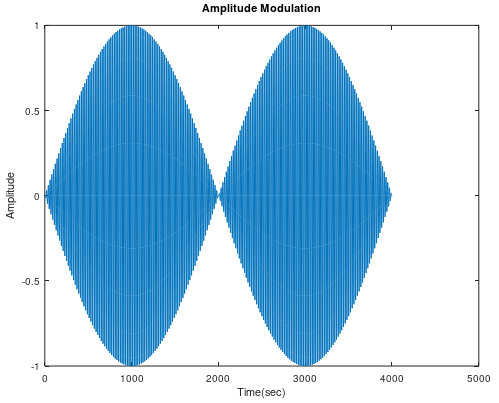# Amplitude Modulation using MATLAB

Amplitude modulation (AM) is a modulation technique utilized in electronic communication, most ordinarily for transmitting data by means of a carrier wave. In amplitude modulation, the amplitude that is the signal quality of the carrier wave differs with respect to that of the message signal being transmitted.

Amplitude modulation in MATLAB can be achieved by using the ammod() function.

## ammod()

Syntax : y = ammod(x, Fc, Fs, ini_phase, carramp)
Parameters :

• x : amplitude signal
• Fc : carrier signal frequency
• Fs : sampling frequency
• ini_phase : initial phase in the modulated signal y in radians
• carramp : carrier amplitude of the modulated signal

Returns : amplitude modulated (AM) signal

Example: Amplitude modulation of a sine wave with only 3 parameters.

## MATLAB

 `% carrier Frequency` `Fc = 200; `   `% sampling frequency` `Fs= 4000;`   `% time Duration` `t = (0 : 1 / Fs : 1); `   `% sine Wave with time duration of 't'` `x = sin(2*pi*t);` ` `  `% Amplitude Modulation` `y = ammod(x, Fc, Fs);`   `plot(y);` `title(``'Amplitude Modulation'``);` `xlabel(``'Time(sec)'``);` `ylabel(``'Amplitude'``);`

Output :Whether you're preparing for your first job interview or aiming to upskill in this ever-evolving tech landscape, GeeksforGeeks Courses are your key to success. We provide top-quality content at affordable prices, all geared towards accelerating your growth in a time-bound manner. Join the millions we've already empowered, and we're here to do the same for you. Don't miss out - check it out now!